Related Articles

# Averaging over every N elements of a Numpy Array

• Difficulty Level : Expert
• Last Updated : 16 May, 2021

In this article, we will learn how to find the average over every n element of a NumPy array. For doing our task, we will some inbuilt methods provided by NumPy module which are as follows:

• numpy.average() to calculate the average i.e the sum of all the numbers divided by the number of elements
• numpy.reshape() to reshape the array taking n elements at a time without changing the original data
• numpy.mean() to calculate the average as mean is nothing but the sum of elements divided by the number of elements

Example 1: Average over a 1-D array

Attention geek! Strengthen your foundations with the Python Programming Foundation Course and learn the basics.

To begin with, your interview preparations Enhance your Data Structures concepts with the Python DS Course. And to begin with your Machine Learning Journey, join the Machine Learning - Basic Level Course

## Python3

 `import` `numpy as np`` ` `# converting list to numpy array``givenArray ``=` `np.array([``6``, ``5``, ``4``, ``3``, ``2``, ``1``, ``9``,``                       ``8``, ``7``, ``12``, ``11``, ``10``, ``15``, ``                       ``14``, ``13``])`` ` `# here we took 3 as our input``n ``=` `3`` ` `# calculates the average``avgResult ``=` `np.average(givenArray.reshape(``-``1``, n), axis``=``1``)`` ` `print``(``"Given array:"``)``print``(givenArray)`` ` `print``(``"Averaging over every "``, n, ``" elements of a numpy array:"``)``print``(avgResult)`

Output: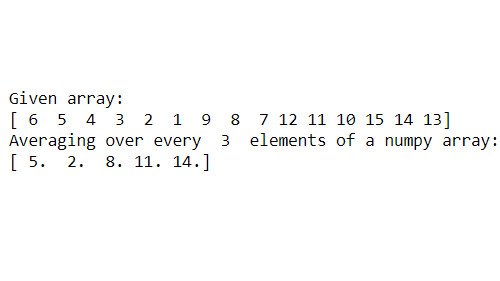Note: N should be an integer multiple of the size of 1d array.

Example 2: Average over a 1-D array(Row-wise)

Here we have taken an array of dimensions (5,3) i.e it has 5 rows and 3 columns. Since the axis=1, it will reshape the elements in groups of n and then calculate the average row-wise using axis=1.

## Python3

 `import` `numpy as np`` ` `# converting list to numpy array``givenArray ``=` `np.array([[``60``, ``50``, ``40``], [``30``, ``20``, ``10``], [``90``, ``80``,``70``],``                       ``[``120``, ``110``, ``100``], [``150``, ``140``, ``130``]])`` ` `# here we took 5 as our input``n ``=` `5`` ` `# calculates the average``avgResult ``=` `np.average(givenArray.reshape(``-``1``, n), axis``=``1``)`` ` `print``(``"Given array:"``)``print``(givenArray, ``"\n"``)`` ` `print``(``"Dimensions of given array:"``, givenArray.shape, ``"\n"``)`` ` `print``(``"Averaging over every "``, n, ``" elements of a numpy array:"``)``print``(avgResult)`

Output: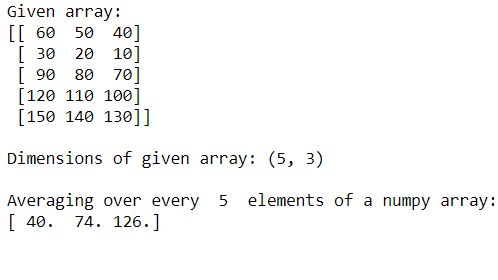Example 3: Average over a 1-D array(Column-wise)

Remember we need to give the axis=1 only then it can group elements row-wise starting from the 0th index. Now if we change the axis value to 0, then after reshaping in groups of n, it will perform the average operation column-wise as given below which will not give us the desired result. It is best if we want to calculate the average column-wise.

## Python3

 `import` `numpy as np`` ` `# converting list to numpy array``givenArray ``=` `np.array([[``60``, ``50``, ``40``], [``30``, ``20``, ``10``], [``90``, ``80``, ``70``],``                       ``[``120``, ``110``, ``100``], [``150``, ``140``, ``130``]])`` ` `# here we will calculate average``# over every 5 elements``n ``=` `5`` ` `# calculates the average``avgResult ``=` `np.average(givenArray.reshape(``-``1``, n), axis``=``0``)`` ` `print``(``"Given array:"``)``print``(givenArray, ``"\n"``)`` ` `print``(``"Dimensions of given array:"``, givenArray.shape, ``"\n"``)`` ` `print``(``"Averaging over every "``, n, ``" elements of a numpy array:"``)``print``(avgResult)`

After reshaping the 2D array it looks like below: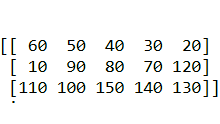Then performing the average column wise we get the answer.

Output: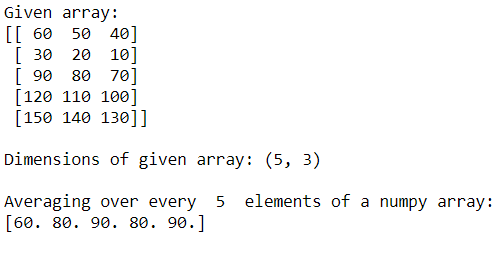Example 4: Average over a 1-D array(Column-wise without reshaping)

Note here that taking axis=0 we cannot perform the average row-wise over every n element. It will just calculate the average of each column separately. The below code will calculate the average over every column element.

## Python3

 `import` `numpy as np``# converting list to numpy array``givenArray ``=` `np.array([[``60``, ``50``, ``40``], [``30``, ``20``, ``10``], [``90``, ``80``,``70``],``                       ``[``120``, ``110``, ``100``], [``150``, ``140``, ``130``]])`` ` `# here we will calculate average over``# every 5 elements``n ``=` `5`` ` `# calculates the average``avgResult1 ``=` `givenArray.mean(axis``=``0``)`` ` `print``(``"Given array:"``)``print``(givenArray, ``"\n"``)`` ` `print``(``"Dimensions of given array:"``, givenArray.shape, ``"\n"``)`` ` `print``(``"Averaging over every "``, n, ``" elements of a numpy array:"``)``print``(avgResult1)`

Output: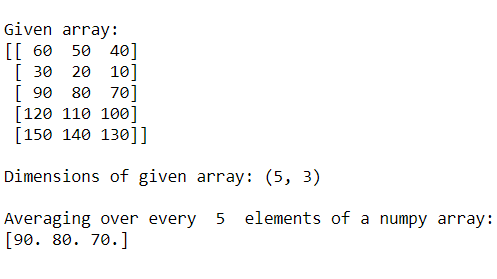My Personal Notes arrow_drop_up